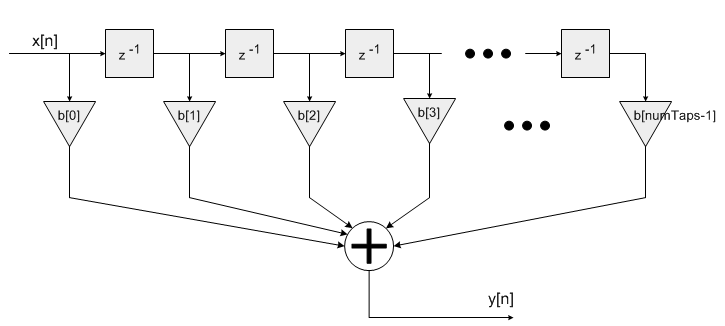Project Ne10 An open, optimized software library for the ARM architecture.
Finite Impulse Response (FIR) Filters

## Functions

ne10_result_t ne10_fir_init_float (ne10_fir_instance_f32_t *S, ne10_uint16_t numTaps, ne10_float32_t *pCoeffs, ne10_float32_t *pState, ne10_uint32_t blockSize)

void ne10_fir_float_c (const ne10_fir_instance_f32_t *S, ne10_float32_t *pSrc, ne10_float32_t *pDst, ne10_uint32_t blockSize)
Specific implementation of ne10_fir_float using plain C. More...

void ne10_fir_float_neon (const ne10_fir_instance_f32_t *S, ne10_float32_t *pSrc, ne10_float32_t *pDst, ne10_uint32_t blockSize) asm("ne10_fir_float_neon")
Specific implementation of ne10_fir_float using NEON SIMD capabilities. More...

## Variables

void(* ne10_fir_float )(const ne10_fir_instance_f32_t *S, ne10_float32_t *pSrc, ne10_float32_t *pDst, ne10_uint32_t blockSize)

## Detailed Description

This set of functions implements Finite Impulse Response (FIR) filters for floating-point data types. The functions operate on blocks of input and output data and each call to the function processes `blockSize` samples through the filter. `pSrc` and `pDst` points to input and output arrays containing `blockSize` values.
Algorithm:
The FIR filter algorithm is based upon a sequence of multiply-accumulate (MAC) operations. Each filter coefficient `b[n]` is multiplied by a state variable which equals a previous input sample `x[n]`.
```     y[n] = b * x[n] + b * x[n-1] + b * x[n-2] + ...+ b[numTaps-1] * x[n-numTaps+1]
```Finite Impulse Response filter
`pCoeffs` points to a coefficient array of size `numTaps`. Coefficients are stored in time reversed order.
```     {b[numTaps-1], b[numTaps-2], b[N-2], ..., b, b}
```
`pState` points to a state array of size `numTaps + blockSize - 1`. Samples in the state buffer are stored in the following order.
```     {x[n-numTaps+1], x[n-numTaps], x[n-numTaps-1], x[n-numTaps-2]....x, x, ..., x[blockSize-1]}
```
Note that the length of the state buffer exceeds the length of the coefficient array by `blockSize-1`. The increased state buffer length allows circular addressing, which is traditionally used in the FIR filters, to be avoided and yields a significant speed improvement. The state variables are updated after each block of data is processed; the coefficients are untouched.
Instance Structure
The coefficients and state variables for a filter are stored together in an instance data structure. A separate instance structure must be defined for each filter. Coefficient arrays may be shared among several instances while state variable arrays cannot be shared. There are separate instance structure declarations for each of the 4 supported data types.
Initialization Functions
There is also an associated initialization function for each data type. The initialization function performs the following operations:
• Sets the values of the internal structure fields.
• Zeros out the values in the state buffer.
Use of the initialization function is optional. However, if the initialization function is used, then the instance structure cannot be placed into a const data section. To place an instance structure into a const data section, the instance structure must be manually initialized. Set the values in the state buffer to zeros before static initialization. The code below statically initializes each of the 4 different data type filter instance structures
```*ne10_fir_instance_f32_t S = {numTaps, pState, pCoeffs};
```

where `numTaps` is the number of filter coefficients in the filter; `pState` is the address of the state buffer; `pCoeffs` is the address of the coefficient buffer.

Fixed-Point Behavior
Care must be taken when using the fixed-point versions of the FIR filter functions. In particular, the overflow and saturation behavior of the accumulator used in each function must be considered. Refer to the function specific documentation below for usage guidelines.

## Function Documentation

 void ne10_fir_float_c ( const ne10_fir_instance_f32_t * S, ne10_float32_t * pSrc, ne10_float32_t * pDst, ne10_uint32_t blockSize )

Specific implementation of ne10_fir_float using plain C.

Definition at line 111 of file NE10_fir.c.

 void ne10_fir_float_neon ( const ne10_fir_instance_f32_t * S, ne10_float32_t * pSrc, ne10_float32_t * pDst, ne10_uint32_t blockSize )

Specific implementation of ne10_fir_float using NEON SIMD capabilities.

 ne10_result_t ne10_fir_init_float ( ne10_fir_instance_f32_t * S, ne10_uint16_t numTaps, ne10_float32_t * pCoeffs, ne10_float32_t * pState, ne10_uint32_t blockSize )
Parameters
 [in,out] *S points to an instance of the floating-point FIR filter structure. [in] numTaps Number of filter coefficients in the filter. [in] *pCoeffs points to the filter coefficients buffer. [in] *pState points to the state buffer. [in] blockSize number of samples that are processed per call.

Description:

`pCoeffs` points to the array of filter coefficients stored in time reversed order:
```   {b[numTaps-1], b[numTaps-2], b[N-2], ..., b, b}
```
`pState` points to the array of state variables. `pState` is of length `numTaps+blockSize-1` samples, where `blockSize` is the number of input samples processed by each call to `arm_fir_f32()`.
Examples:
NE10_sample_fir.c.

Definition at line 53 of file NE10_fir_init.c.

## Variable Documentation

 void(* ne10_fir_float) (const ne10_fir_instance_f32_t *S, ne10_float32_t *pSrc, ne10_float32_t *pDst, ne10_uint32_t blockSize)
Parameters
 [in] *S points to an instance of the floating-point FIR filter structure. [in] *pSrc points to the block of input data. [out] *pDst points to the block of output data. [in] blockSize number of samples to process per call.

Points to ne10_fir_float_c or ne10_fir_float_neon.

Examples:
NE10_sample_fir.c.

Definition at line 163 of file NE10_init_dsp.c.